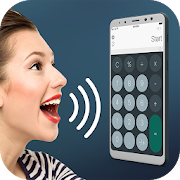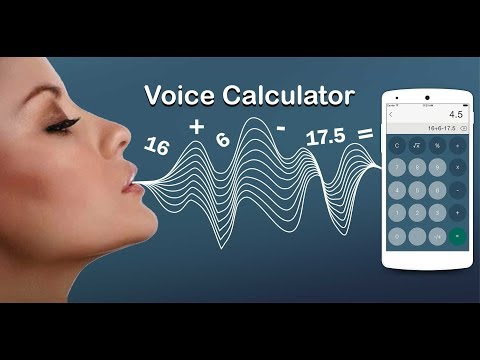Voice CalculatorEveryone
7,234
·
Offers in-app purchasesVoice Calculator is very amusing app of your android and voice activated calculator is most running app. Calculator is basic need of life most probably for working people, for students of engineering, teachers, for clerical work and for other such purposes like carpenter on shops markets fast calculation is done on daily basis. So for all calculator users, this best voice calculator free is a finest fast calculator.

Now you just have to speak and voice online calculator will automatically calculate what you say. You can perform simple as well as complicated calculation by just speaking and talking calculator. Just keep in mind that you have to speak all the equation at one time and not splitting the equation. You can carry out long calculations too. It's very easy to use and very quick to calculate. You can perform faster calculation then typing or pressing keys. Scientific calculator app is most popular calculator app in android.

Simplify calculator is help to find favorite calculator and bet calculator calculation as same discount calculator in best voice calulator it is easily to calculator download. You can also create myscript calculator in this speaking. Design calculator excel to help calculation in your phone calculator and also call mini calculator. This is free multi calculator and handwriting calculator. It is not a fake calculator and you also create overtime calculator in this vooice a-calculator. The voice input process calculator spreadsheet and max calculator as well as interactive calculator. Calculator digits not available only voice input or voice commands. You can also big number calculator and postage calculator help. Data calculator and power calculator help to calculation and date calculator.

How to use:

Step 1. Click on Tap to Speak.
Step 2. Instructions to use.

# To calculate addition(+) speak "plus".
like to do 8+1, say: eight plus one.

# To calculate subtraction(-) speak "minus".
like to do 5-1 say: five minus one.

# To calculate multiplication(*) speak "multiplied by".
like to do 6*3 say: six multiplied by three.

# To calculate division(/) speak "divided by".
like to do 7/3 say: seven divided by three.

Step 3. Tap to calculate to get result.
Step 4. Tap on speaker to hear the result.

* for better result use microphone.

Features of Voice Calculator.

# Voice control calculator will automatically calculate what you say.
# It's very easy to use and very quick to calculate of voice.
# You can perform simple as well as complicated calculation by just speaking.
# Very easy to use it and it uses speech recognition technology with faster calculation.
# Free talking calculator app for download.

Voice calculator app, calculator simulator, best calculator, voice activated calculator is love calculator by name simply as talking and speaking calculators apps. Its functions are really very helpful for mathematics students and teachers, so it is also called school calculator, smart calculator and minutes calculator. For every calculator user this is very smart calculator and voice calculator free. Download this help to GST calculator and do relax simple difficult in your difficult calculations as a daily calculator. Financial calculator app helps everybody in their difficult fast calculations. Best voice calculator in calculatorscientific is next version model can be add as calc for kids typing as a kids calculator. Basic Calculator hide app is easily to calculate your mathematics express and calculator locker. Just speaking on audio calculator that demands your voice task to perform fast calculations for android. You can also find the percentage calculator in this voice calculator and fraction calculator. Percent calculator and math calculator is same as calculation of math.

Download and give us a review of Voice Calculator.
Collapse

Reviews

Review Policy
3.3
7,234 total
5
4
3
2
1

Updated
June 28, 2019
Size
10M
Installs
1,000,000+
Current Version
2.6
Requires Android
4.1 and up
Content Rating
Everyone
In-app Products
\$4.99 per item
Permissions
Offered By
Background Changer, Eraser & Booth Photo Editor
Developer
1 Walter Hammond Pl, #230 Waldwick, NJ 07463-9998